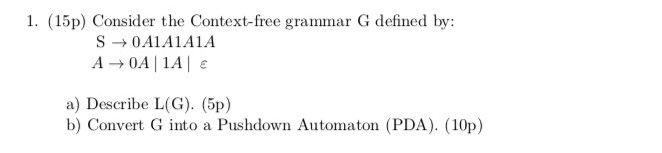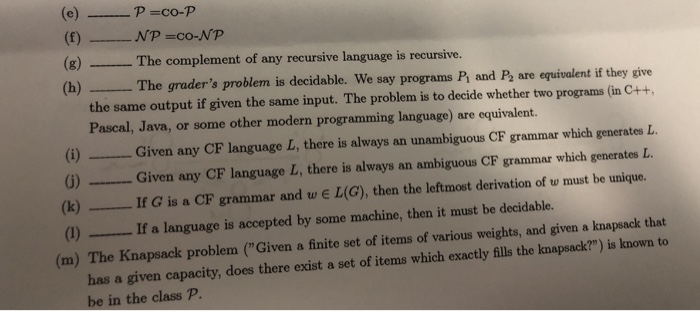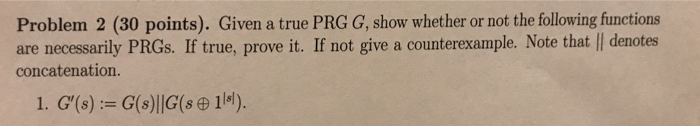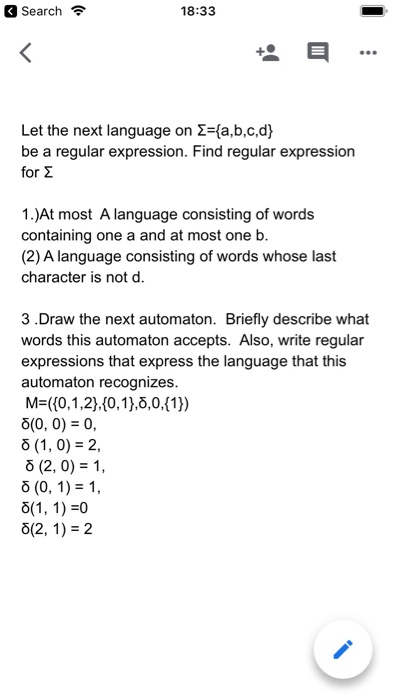# If L is recursive, is it necessarily true that L^+ is also recursive? Peter Lin: Formal...

If L is recursive, is it necessarily true that L^+ is also recursive?

Peter Lin: Formal Language and Automata

Recursive languages are closed under the following operations. That is, if L and P are two recursive languages, then the following languages are recursive as well :

The concatenation L\circ P
The union L\cup P
The intersection L\cap P
The complement of L
The Kleene closure or Kleene star L*
The Kleene Plus L+ (Which You were asking)
The set difference L-P
The last property follows from the fact that the set difference can be expressed in terms of intersection and complement.

"Every Language type which is closed under Kleene star, is also closed under Kleene Plus.

Every Language type which is NOT closed under Kleene star, is also NOT closed under Kleene Plus. "

#### Earn Coin

Coins can be redeemed for fabulous gifts.

Similar Homework Help Questions
• ### Is it true that every recursively enumerable language is recursive, and is it true that every...

Is it true that every recursively enumerable language is recursive, and is it true that every language is recursively enumerable?

• ### Let L be a recursive language. Define L' = {x : there is y such that...

Let L be a recursive language. Define L' = {x : there is y such that yxy belongs to L. Show that L' is r.e.

• ### formal language automata 1. (15p) Consider the Context-free grammar G defined by: S → 0A1A1A1A A0A1A...formal language automata 1. (15p) Consider the Context-free grammar G defined by: S → 0A1A1A1A A0A1A a) Describe L(G). (5p) b) Convert G into a Pushdown Automaton (PDA). (10p)

• ### true or False with prove? (f) ___ NP =co-NP (g) The complement of any recursive language...true or False with prove? (f) ___ NP =co-NP (g) The complement of any recursive language is recursive. h) The grader's problem is decidable. We say programs Pi and P are equivalent if they give the same output if given the same input. The problem is to decide whether two programs (in C++, Pascal, Java, or some other modern programming language) are equivalent. )Given any CF language L, there is always an unambiguous CF grammar which generates L 6)Given any...

• ### Construct a NPDA with transition graph using 4 states that accepts the language L={w: na(w)-nb(w)=2} on...

Construct a NPDA with transition graph using 4 states that accepts the language L={w: na(w)-nb(w)=2} on Σ={a,b} subject-- formal language of automata theory.

• ### Formal languages and automata: Give a regular expression for L={anbm:n?2,m?1,nm?3}

Formal languages and automata: Give a regular expression for L={anbm:n?2,m?1,nm?3}

• ### 14.) Let L1 = L(a∗baa∗) and L2 = L(aba∗). Find L1/L2. This is a Formal Languages and Automata que...

14.) Let L1 = L(a∗baa∗) and L2 = L(aba∗). Find L1/L2. This is a Formal Languages and Automata question... I need to see what the dfa for L1/L2 looks like and how you know what the final states are... Thanks!

• ### Given a true PRG G, show whether or not the following functions are necessarily PRGs. If true, pr...Given a true PRG G, show whether or not the following functions are necessarily PRGs. If true, prove it. If not give a counterexample. Note that jj denotes concatenation G'(s) : = G (s) II G (s    1 IsI  )   Problem 2 (30 points). Given a true PRG G, show whether or not the following functions are necessarily PRGs. If true, prove it. If not give a counterexample. Note that l| denotes concatenation. Problem 2 (30 points). Given a true...

• ### FORMAL LANGUAGE AUTOMATA please wxplain and draw thanks Search令 18:33 Let the next language on Σ-{a,b,c,d)...FORMAL LANGUAGE AUTOMATA please wxplain and draw thanks Search令 18:33 Let the next language on Σ-{a,b,c,d) be a regular expression. Find regular expression for Σ 1.)At most A language consisting of words containing one a and at most one b (2) A language consisting of words whose last character is not d. 3.Draw the next automaton. Briefly describe what words this automaton accepts. Also, write regular expressions that express the language that this automaton recognizes. M-(10,1,2),10,13,0,0,11) 5(0, 0) 0, 82,...